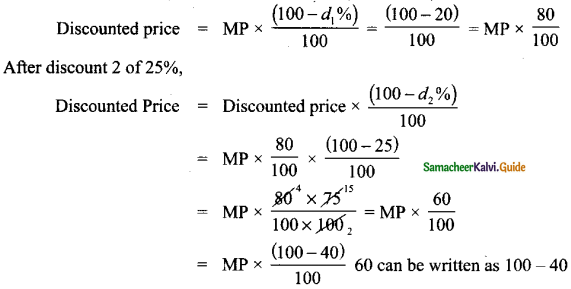Tamilnadu State Board New Syllabus Samacheer Kalvi 8th Maths Guide Pdf Chapter 4 Life Mathematics Ex 4.4 Text Book Back Questions and Answers, Notes.

## Tamilnadu Samacheer Kalvi 8th Maths Solutions Chapter 4 Life Mathematics Ex 4.2

Question 1.
Fill in the blanks:
(i) Loss or gain percentage is always calculated on the ________ .
Cost Price

(ii) A mobile phone is sold for ₹ 8400 at a gain of 20%. The cost price of the mobile phone is ________ .
₹ 7000
Hint:
Let cost price of mobile be ₹ x
Given that selling price is ₹ 8400 and gain is 20%
As per formula,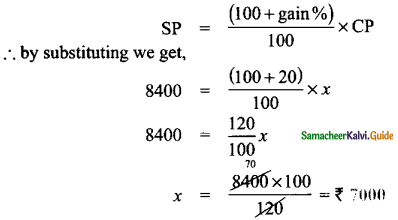(iii) An article is sold for ₹ 555 at a loss of 7$$\frac { 1 }{ 2 }$$%. The cost price of the article is ________ .
₹ 600
Hint:
Given selling price is ₹ 555 & loss 7$$\frac { 1 }{ 2 }$$%
as per formula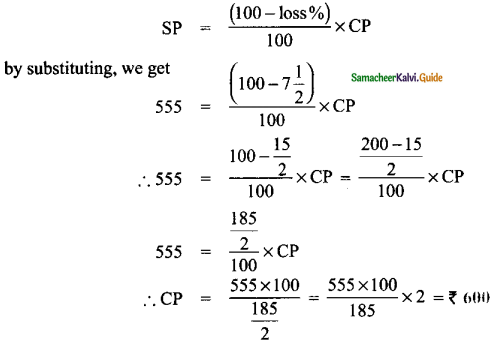(iv) A mixer grinder marked at ₹ 4500 is sold for ₹ 4140 after discount. The rate of discount is ________ .
8 %
Hint:
Marked price is ₹ 4500
Discounted price in ₹ 4140
∴ Discount = Marked price – Discounted priòe
= 4500 – 4140 = 360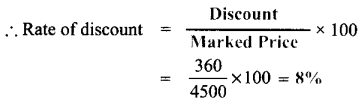(v) The total bill amount of a shirt costing ₹ 575 and a T-shirt costing ₹ 325 with GST of 5% is ________ .
Cost of price shirt = ₹ 575 (CP)
GST = 5%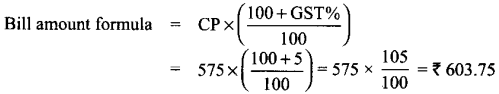Cost of price shirt = ₹ 325 (CP)
GST = 5%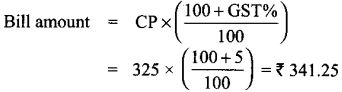∴ Total bill amount = ₹ 603.75 + ₹ 341.25 = ₹ 945

Question 2.
If selling an article for ₹ 820 causes 10% loss on the selling price, then find its cost price.
Given that selling price (SP) = ₹ 820
Loss % = 10 %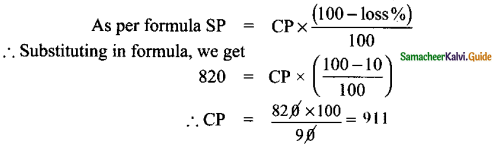Question 3.
If the profit earned on selling an article for ₹ 810 is the same as loss on selling it for ₹ 530, then find the cost price of the article.
Case 1: Profit = Selling price (SP) – Cost price (CP)
Case 2: Loss = Cost price (CP) – Selling price (SP)
Given that profit of case 1 = loss of case 2
∴ P = 810 – CP
L = CP – 530
Since profit (P) = loss (L)
810 – CP = CP – 530
∴ 2CP = 810 + 530 = 1340 ⇒ C.P = $$\frac{1340}{2}$$
∴ CP = 670Question 4.
If the selling price of 10 rulers is the same as the cost price of 15 rulers, then find the profit percentage.
Let cost price of one ruler be x
Given that selling price (SP) of 10 rulers.
i.e., same as cost price (CP) of 15 rulers
∴ SP of 10 rulers = 15 × x = 15x
∴ SP of 1 ruler = $$\frac{15 x}{10}$$ = 1.5x
∴ Gain = SP of 1 ruler – CP of 1 ruler = 1.5x – x = 0.5x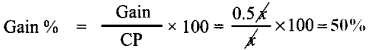Question 5.
Some articles are bought at 2 for ₹ 15 and sold at 3 for ₹ 25. Find the gain percentage.
Let cost price of one article be C.P
Given that 2 are bought for ₹ 15
∴ 2 × CP = 15 ⇒ CP = $$\frac{15}{2}$$
Let selling price of one article be SP
Given that 3 are sold for ₹ 25
∴ 3 × SP = 25 ⇒ SP = $$\frac{25}{3}$$
∴ Gain = SP – CP = $$\frac{25}{3}-\frac{15}{2}=\frac{50-45}{6}=\frac{5}{6}$$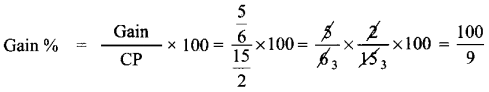= $$11 \frac{1}{9}$$Question 6.
By selling a speaker for ₹ 768, a man loses 20%. In order to gain 20%, how much should he sell the speaker?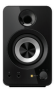Selling price (SP) of speaker = ₹ 768
Loss % = 20 %
as per formula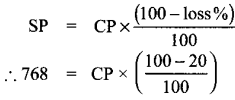∴ CP = $$\frac{768 \times 100}{80}$$ = 960
For gain of 20%, we should now calculate the selling price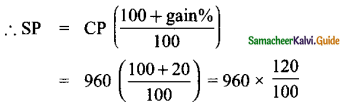= 96 × 12 = ₹ 1152

Question 7.
Find the unknowns x, y and z.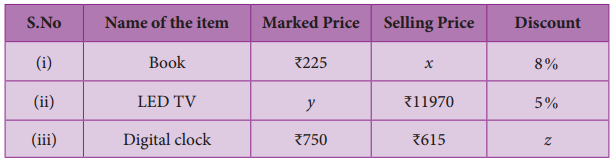(i) Book marked price = ₹ 225 discount 8%
∴ Selling price (x) = Marked price × $$\left(\frac{(100-d \%)}{100}\right)$$
= 225 × $$\frac{(100-8)}{100}$$ = 225 × $$\frac{92}{100}$$ = ₹ 207

(ii) LED TV selling price = 11970 discount = 5%, Marked price = y
∴ Selling price Marked price y × $$\left(\frac{(100-d \%)}{100}\right)$$
∴ 11970 = y × $$\frac{(100-5)}{100}$$
∴ y = $$\frac{11970 \times 100}{95}$$ = 126 × 100 = ₹ 12,600

(iii) Digital clock marked price (MP) = ₹ 750, MP = ₹ 12.600
Selling price (SP) = ₹ 615, Discount = z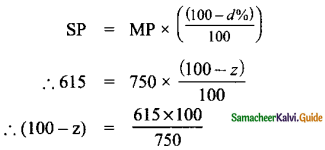100 – z = 82
∴ z = 100 – 82, Discount = 18%Question 8.
Find the total bill amount for the data given below: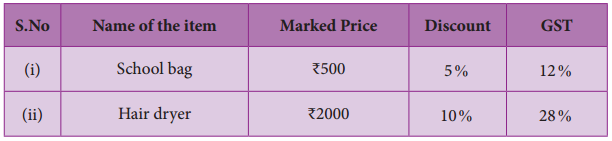Formula for discounted price LW = Marked price (MP) × $$\frac{(100-d \%)}{100}$$
When d is the discount %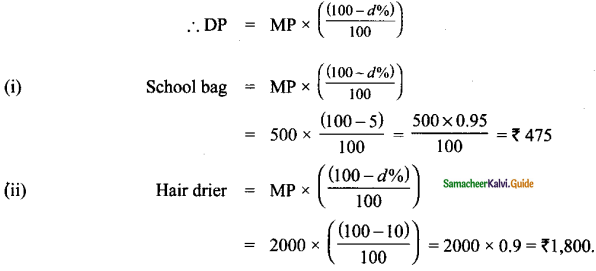For bill amount, we should apply GST on the discounted value of the items.
Formula: Bill amount = Discounted price × $$\left(\frac{(100+\mathrm{GST} \%)}{100}\right)$$
∴ For (i) School bag.
Bill amount 475 × $$\left(\frac{(100+12)}{100}\right)$$ = 475 × 1.12 = %‘532
∴ For (ii) Hair drier,
Bill amount = 1800 × $$\left(\frac{(100+28)}{100}\right)$$ = 1800 × 1.28 =
∴ Total bill amount Bill amount of School bag + Stationary + Cosmetics + Hair drier
= 532 + 252 + 1357 + 2304
= ₹ 4.445Question 9.
A branded Air-Conditioner (AC) has a marked price of ₹ 38000. There are 2 options given for the customer.
(i) Selling Price is the same ₹ 38000 but with attractive gifts worth ₹ 3000
(or)
(ii) Discount of 8% on the marked price but no free gifts. Which offer is better?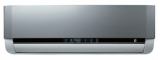Marked price of AC = ₹ 38,000
Option 1:
Selling price = ₹ 38000 & gifts worth ₹ 3000
∴ Net gain for customer = ₹ 3000 as there is no discount on AC

Option 2:
Discount of 8%, but no gift
∴ Discounted value = MP × $$\left(\frac{(100-d \%)}{100}\right)$$
38000 × $$\frac{(100-8)}{100}$$ = 38000 × 0.92 = 34960
∴ Savings for customer = 38000 – 34960 = 3040
Therefore, the customer gets 3000 gift in option I where as he is able to save only ₹ 3040 in option 2. Therefore, option 2 is better.

Question 10.
If a mattress is marked for ₹ 7500 and is available at two successive discount of 10% and 20%, find the amount to be paid by the customer.
Marked price of mattress = ₹ 7500
Discount d1 = 10%
Discount d2 = 20%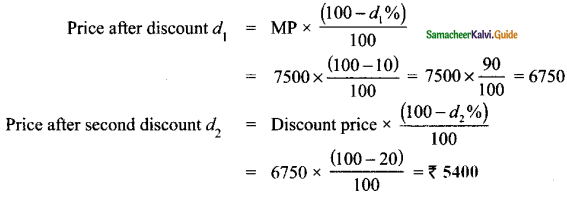Objective Type Questions

Question 11.
A fruit vendor sells fruits for ₹ 200 gaining ₹ 40. His gain percentage is
(A) 20%
(B) 22%
(C) 25%
(D) 16
(C) 25%
Hint:
Selling price ₹ 200
Gain = 40
∴ CP – Selling price – gain = 200 – 40 = 160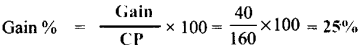Question 12.
By selling a flower pot for Z528, a woman gains 20%. At what price should she sell it to gain 25%?
(A) ₹ 500
(B) ₹ 550
(C) ₹ 553
(D) ₹ 573
(B) ₹ 550
Hint:
If selling price (sp) = ₹ 528
Gain % = 20 %
∴ CP = ?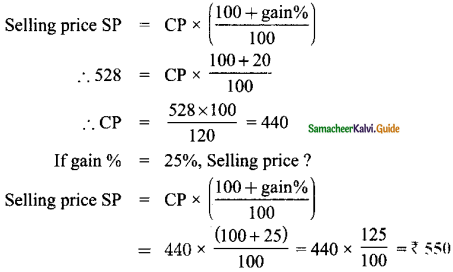Question 13.
A man buys an article for ₹ 150 and makes overhead expenses which are 12% of the cost price. At what price must he sell it to gain 5%?
(A) ₹ 180
(B) ₹ 168
(C) ₹ 176.40
(D) ₹ 88.20
(C) ₹ 176.40
Hint:
Cost price of article = ₹ 150
Over head expenses = 12% of cost price
= $$\frac{12}{100}$$ × 150 = ₹ 18
∴ Effective cost of article = 150 + 18 = ₹ 168
Now, to gain 5%, he has to sell at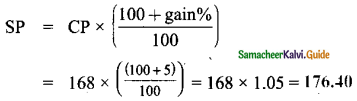Question 14.
What is the marked price of a hat which is bought for Z210 at 16% discount?
(A) ₹ 243
(B) ₹ 176
(C) ₹ 230
(D) ₹ 250
(D) ₹ 250
Hint:
Let marked price be MP
Discounted price = ₹ 210
Rate of discount = 16%
As per formula: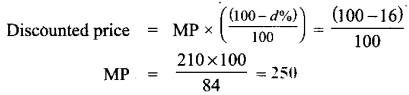Question 15.
The single discount in % which is equivalent to two successive discounts of 20% and 25% is
(A) 40%
(B) 45%
(C) 5%
(D) 22.5%